# AP Calculus Review: Reasoning with Theorems and Definitions

Many people believe that mathematics is about number-crunching, but much more importantly, math is about reasoning. For example, when you solve a word problem, you are using your reasoning skills to put together the given information in just the right way.

In a way, AP Calculus is all about reasoning. You have to interpret each problem and correctly apply the appropriate methods (limits, derivatives, integrals, etc.) to solve it. However sometimes we have to take it one step further and reason with theorems and definitions as well, gluing our thoughts together with mathematical logic.

Why is this important? Well using nothing more than a handful of assumptions and plenty of definitions, theorems, and logic, Euclid developed the entire subject of Geometry from the ground up! If that’s not a reason to respect the power of definitions and theorems, then nothing else is.## What are Definitions and Theorems?

In mathematics, every term must be defined in some way. A definition of a mathematical object is formal description of the essential properties that make that object what it is. For instance,

• Definition: A triangle is a three-sided polygon.

It’s very important to understand the definitions of our mathematical terms so that we can employ just the right tool in each specific case. So if you see a three-sided polygon in a problem, then you know that it’s a triangle by definition. Then you may use a property or formula related to triangles as part of your reasoning steps.

We also rely on general statements of truth called theorems in order to reason about a specific situation. Speaking of triangles, perhaps one of the most famous (and useful) theorems of all time is the Pythagorean Theorem. (By the way, this theorem shows up in Book 1 of Euclid’s Elements, over 2000 years ago!)Diagram for Pythagoras theorem by Drini (Pedro Sanchez)

## Reasoning with Definitions

On the AP Calculus exams, you must know and be able to apply the definitions of calculus. Let’s see what that means in an example problem.

### Example 1

If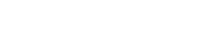, which of the following is true?

(A) f(x) is continuous and differentiable at x = 3

(B) f(x) is continuous but not differentiable at x = 3

(C) f(x) is neither continuous nor differentiable at x = 3

(D) f(x) is differentiable but not continuous at x = 3

In order to properly address this question, we must know the definitions of continuous and differentiable.

• A function f is continuous at a point x = a if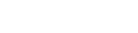• A function f is differentiable at a point x = a if the derivative f ‘(a) exists.

#### Example Solution — Continuity

First let’s determine if the function is continuous at x = 3. Because f is defined piece-wise, we must compute both the left and right hand limits.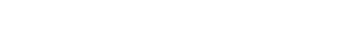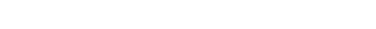Now because the left and right hand limits agree, we know that the two-sided limit as x → 3 exists and equals 0. Next, check the function value at x = 3.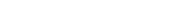Therefore, since the limiting value equals the function value (both are 0), the function f is continuous at x = 3 by definition. (For more about this topic, check out AP Calculus Exam Review: Limits and Continuity.)

#### Example Solution — Differentiability

Moving on to differentiability, now we must check whether f ‘(3) exists. Again, because f is defined piece-wise, we must be careful at the point where the function changes behavior. First find the derivative of each piece.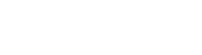Note, there is no typo here — the derivative of the first piece can only be found when x < 3. In fact it takes more analysis to figure out what happens at x = 3.

Because the derivative itself is actually a certain kind of limit (by definition!), we’ll have to see what the limiting values for f ‘ are as x → 3. As before, examine each piece separately.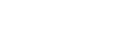This time there is a mismatch. Because the left and right derivatives do not agree (18 ≠ -9), the derivative does not exist at x = 3. Thus by definition, f is not differentiable at x = 3.

In summary, f is continuous, but not differentiable at x = 3. Choice (B) is correct.

## Reasoning with Theorems

Remember, a theorem is a true mathematical statement. Typically theorems are general facts that can apply to lots of different situations. Here is a small list of important theorems in calculus.

### A Theorem by any other Name…

There are many other results and formulas in calculus that may not have the title of “Theorem” but are nevertheless important theorems. Every one of your derivative and antidifferentiation rules is actually a theorem. Here is a partial list of other theorems that may not be explicitly identified as theorems in your textbook.

• Differentiability implies continuity
• The first derivative rule for increase and decrease
• The second derivative rule for concavity
• First and second derivative rules for relative extrema
• Product Rule, Quotient Rule, Chain Rule, etc.
• L’Hospital’s Rule
• Additivity and linearity of the definite integral
• Techniques of antidifferentiation such as substitution, integration by parts, etc.
• Various tests for convergence of series

Now let’s see if we can use the right theorems to crack the next example.

### Example 2

Find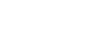.

Notice that this is a derivative of an integral. That means we may be able to apply the Fundamental Theorem of Calculus. There are two parts to the theorem, but the one we need is: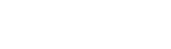However, before we can apply this theorem, we must change the form of the integral. The theorem requires that the lower limit of integration must be a constant. By using the rule for switching the order of integration (another theorem!), we may write:Next, because the upper limit of integration is not a simple variable, x, we must use yet another theorem: the Chain Rule. Here, the “inside function” is u = x3.It’s interesting to note in this case that no other method could have led to the solution. It is impossible to write down an antiderivative for the function, sin t2. Fortunately the Fundamental Theorem of Calculus in the form we used it avoids the antidifferentiation step altogether.

## Conclusion

Definitions and theorems form the backbone of mathematical reasoning. Knowing your definitions means knowing which tools can apply in each situation. And by understanding the theorems, you can avoid doing a lot of unnecessary or difficult work.

#### Improve your SAT or ACT score, guaranteed. Start your 1 Week Free Trial of Magoosh SAT Prep or your 1 Week Free Trial of Magoosh ACT Prep today!## Author

•Shaun earned his Ph. D. in mathematics from The Ohio State University in 2008 (Go Bucks!!). He received his BA in Mathematics with a minor in computer science from Oberlin College in 2002. In addition, Shaun earned a B. Mus. from the Oberlin Conservatory in the same year, with a major in music composition. Shaun still loves music -- almost as much as math! -- and he (thinks he) can play piano, guitar, and bass. Shaun has taught and tutored students in mathematics for about a decade, and hopes his experience can help you to succeed!